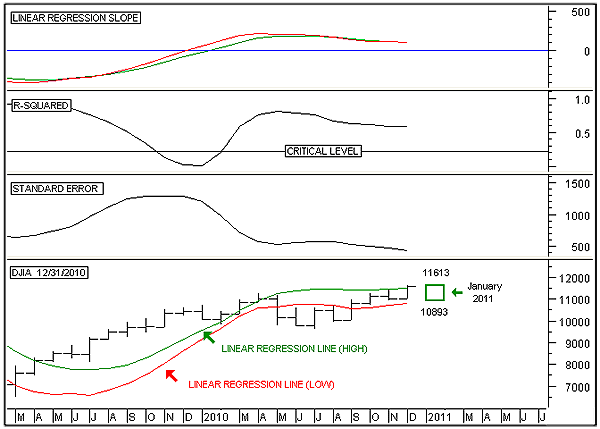HOT TOPICS LIST

INDICATORS LIST

LIST OF TOPICS

# As January Goes

01/04/11 10:24:32 AM
by Alan R. Northam

As January goes, so goes the year. Here I use statistical analysis to forecast what the stock market will do in January and the rest of the year.

Security:   .DJI
Position:   N/A

 The January barometer, devised by Yale Hirsch in 1972, states that as January goes, so goes the year. This barometer of the stock market has only been wrong six times since 1950 for a 90% accuracy ratio. The last time the January barometer was wrong was 2010, when January registered a loss, but the Dow Jones Industrial Average (DJIA) registered an 11% gain. Since the barometer has never been wrong two years in a row, there now exists a high probability that as January 2011 goes, so goes the rest of the year. In this analysis I use statistical analysis to predict what January 2011 will do, which then also predicts what the rest of the year will do. In the following analysis I am using the monthly time frame of the DJIA as shown in Figure 1. To start the prediction, I first look for a time frame in which the standard error indicator is low and pointing lower. Low standard errors means more accurate predictions, whereas high standard errors translate into larger errors in predictions. By plotting the standard error indicator and changing its time period from 10 periods working my way upward, I found that 18 periods gave the lowest standard error and the slope is still pointing lower. This low standard error translates into small deviations in price around the linear regression line.FIGURE 1: DJIA, MONTHLY. This chart shows the monthly price chart of the Dow Jones Industrial Average in the bottom panel, the linear regression slope indicator in the top panel, the R-squared indicator in the second panel, and the standard error indicator in the third. Graphic provided by: MetaStock. Next, I want to check the strength of the trend. To do this, I plot an R-squared indicator just above the standard error indicator setting its time frame to 18. The higher the R-squared value, the more accurately price is following the linear regression line. Here I see that the R-squared indicator is well above the critical level of 0.22, indicating that price is closely following the linear regression line.Then I want to determine the slope of the linear regression line. I do this by monitoring the linear regression slope indicator. Here I plot two linear regression slope indicators. The green line represents the linear regression slope using the high price of the DJIA each month over the past 18 months. The value of this slope at the right edge of the chart is 102. This means that each month over the last 18 months, the linear regression line moved upward by an average of 102 points each month. The red line represents the linear regression slope using the low price of each month over the past 18 months. The value of the slope at the right edge of the chart is also 102, indicating that both the high price and the low price of each month has been rising at the same average slope over the last 18 months. I use the high and low linear regression slopes to determine the high and low price range over the next month.After that, I plot two linear regression lines on the price chart. The green line represents the linear regression line of the high prices at the right edge of the chart over the last 18 months. The value of the green linear regression line at the right edge of the chart is 11511. To plot where the high price is expected to fall one month into the future, I simply add 102 to 11511 to arrive at a high price of 11613 one month into the future. The red line represents the linear regression line of the low prices over the last 18 months. The value of the red linear regression line at the right edge of the chart is 10791. To plot where the low price is expected to fall one month into the future, I simply add 102 to 10791 to arrive at a low price of 10893 one month into the future. This high and low price range over the next one month is then plotted on the chart. In conclusion, this analysis gives a high and low price expectation for the DJIA over the next month. Through this analysis it is expected that January will be a flat month, which in turn indicates that the Dow will end the year close to where it started. However, a close above the expected price range for January would indicate a higher price for 2011, whereas a close below the expected price range will indicate lower prices for the year. Whether the year ends where it starts or if the market ends higher or lower, there is sure to be some great trading opportunities during 2011. Note: A prediction is just that, a prediction. It is a forecast of what the stock market is expected to do in the future and is never to be considered a guarantee.

Alan R. Northam

Alan Northam lives in the Dallas, Texas area and as an electronic engineer gave him an analytical mind from which he has developed a thorough knowledge of stock market technical analysis. His abilities to analyze the future direction of the stock market has allowed him to successfully trade of his own portfolio over the last 30 years. Mr. Northam is now retired and trading the stock market full time. You can reach him at inquiry@tradersclassroom.com or by visiting his website at http://www.tradersclassroom.com. You can also follow him on Twitter @TradersClassrm.

 Comments or Questions? Article Usefulness 5 (most useful) 4 3 2 1 (least useful)